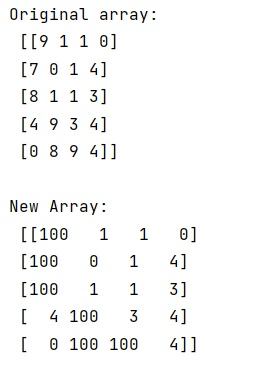# Replacing NumPy elements if condition is met

Learn, how to replace numpy elements if a certain condition is met?
Submitted by Pranit Sharma, on January 06, 2023

NumPy is an abbreviated form of Numerical Python. It is used for different types of scientific operations in python. Numpy is a vast library in python which is used for almost every kind of scientific or mathematical operation. It is itself an array which is a collection of various methods and functions for processing the arrays.

## NumPy - Replacing elements if condition is met

Suppose we are given a NumPy array that we need to manipulate so that each element is changed to either a 1 or 0 if a condition is met.

For this purpose, we will assign a specific value to those positions where the condition is met.

Suppose we need to filter those values which are less than 5, if the condition is met, we will assign it a value (say 100).

Let us understand with the help of an example,

## Python code to replace NumPy elements if condition is met

```# Import numpy
import numpy as np

# Creating a numpy array
arr = np.random.randint(0, 10, size=(5, 4))

# Display original array
print("Original array:\n",arr,"\n")

# Replacing arrays
arr[arr > 5] = 100

# Display result
print("New Array:\n",arr)
```

Output: